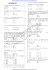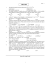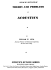# Departmental Practice Final Version 2 15400

## Transcription

Departmental Practice Final Version 2 15400
```MATH 15400 Practice Final (Version #2)
TEXTBOOK: Swokowski & Cole, Algebra & Trigonometry with Analytic Geometry, Classic 12th Edition
answers for errors. Problems involving units must have the units represented on the answer to receive full credit.
1.
Find an equation of the parabola with vertex V (3,  5) and directrix x  2 .
(11.1 #23)
1.
2.
(4)
Find the vertices and the foci of the ellipse. Sketch its graph.
4 x 2  9 y 2  32 x  36 y  64  0
(11.2 #11)
(4)
y
x
3.
Find an equation of the hyperbola that has its center at the origin with vertices V (4, 0)
and passing through the point (8, 2) .
(11.3 #26)
3.
MATH 15400 Practice Final Version 2
Page 1 of 8
(4)
4.
Solve the system.
(9.1 #17)
 x 2  y 2  16

 2 y  x  4
4.
5.
Planning production A small furniture company manufactures sofas and recliners. Each sofa requires 8
hours of labor and \$180 in materials, while a recliner can be built for \$105 in 6 hours. The company has
340 hours of labor available each week and can afford to buy \$6750 work of materials. How many
recliners and sofas can be produced if all labor hours and all materials must be used?
(9.2 #37)
5.
6
(4)
(4)
a) Calculate the length of arc that subtends a central angle of measure   50 on a circle of diameter
16 meters.
(6.1 #35)
6a)
(4)
6b)
(4)
b) Find the area of the sector determined by  in part (a).
MATH 15400 Practice Final Version 2
Page 2 of 8
7.
A wheel of radius 9 inches is rotating at a rate of 2400 rpm.
a) Find the angular speed (in radians per minute).
(6.1 #46)
7a)
(4)
7b)
(4)
b) Find the linear speed of a point on the circumference (in ft/min).
8.
Find the exact values of sin  , cos and tan  if  is in standard position and the terminal side of  is
in quadrant III and parallel to the line 2 y  7 x  2  0 .
(6.2 #79)
8.
9.
(4)
Approximate, to the nearest 0.01 radian, all angles  in the interval [0, 2 ) that satisfy the equation.
(6.4 #37f)
csc   4.8521
9.
MATH 15400 Practice Final Version 2
Page 3 of 8
(4)
10. Graph at least one complete period of y  1  tan x .
(6.3 #59)
(4)
y
x
11. Find the amplitude, period, phase shift, and graph at least one complete period for y  sin 2 x     1 .
(6.5 #13)
(4)
y
x
12. Given the indicated parts of triangle ABC with   90 , express the third part in terms of the first two.
(6.7 #21)
 , a; c
12.
MATH 15400 Practice Final Version 2
Page 4 of 8
(4)
13. An airplane takes off at a 10 angle and travels at the rate of 250 ft/sec. Approximately how long does it
take the airplane to reach an altitude of 15,000 feet?
(6.7 #32)
13.
14. Verify the identity. Show all work.
(4)
(7.1 #9)
(4)
1
1

 2 csc 2 
1  cos  1  cos 
15. Find the exact values of the solutions of the equation that are in the interval [0, 2 ) .
(7.2 #55)
2 tan t  sec 2 t  0
15.
MATH 15400 Practice Final Version 2
Page 5 of 8
(4)
16. If sin    4 and sec   5 for a third quadrant angle  and a first quadrant angle  , find the exact
5
3
value for:
a)
b)
(7.3 #21)
sin(    )
16a)
(4)
16b)
(4)
tan(    )
17. Given sin    45 ; 270    360
MATH 15400 Practice Final Version 2
find the exact value of sin 2 , cos 2 and tan 2 .
Page 6 of 8
(7.4 #4)
17a) sin 2 :
(4)
17b) cos 2 :
(4)
17c) tan 2 :
(4)
18. Find the exact values of the solutions of the equation that are in the interval 0, 2  .
(7.4 #37)
cos u  cos 2u  0
18.
19. Without using your calculator, find the exact value of the expression, if it is defined.

(4)
(7.6 #15b)
 
sec tan 1  53
19.
(4)
20. Use inverse trigonometric functions to find the solutions of cos2 x  2 cos x  1  0 that are in 0, 2  , and
approximate the solutions to four decimal places.
(7.6 #53)
20.
MATH 15400 Practice Final Version 2
Page 7 of 8
(4)
21. A forest ranger at an observation point A sights a fire in the direction N 2710E . Another ranger at an
observation point B, 6.0 miles due east of A, sights the same fire at N 5240W . Approximate the distance
from observation point A to the fire.
(8.1 #25)
21.
22. In triangle ABC if   11510, a  1.10 and b  2.10 find the value of side c.
22.
(4)
(8.2 #9)
(4)
23. Use Heron's formula to approximate the area of triangle ABC.
a  25.0 ft , b  80.0 ft , c  60.0 ft
(8.2 #39)
23.
Bonus: Find the exact values of the solutions of the equation that are in the interval 0, 2  .
(4)
(7.2 #39)


cos 2 x    0
4

Bonus:
MATH 15400 Practice Final Version 2
Page 8 of 8
(4)
```

### Document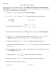### Calculus I Final, Sample### Higher Relationships and Calculus NAB Revision Pack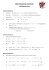### Document 6539622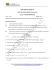### Mathematics - Paper - II (Set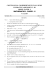### Chapter 6 Problems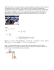### Sample Solutions to Quiz 3 for MATH3270A − y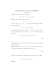### AS Entrance Examination Sample Paper Mathematics Instructions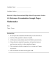### CLASS 12 MATHEMATICS SAMPLE PAPER – 1 SECTION – A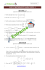### For B.Tech. Physics 20 Chemistry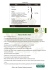### MATH 307: Problem Set #6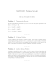### File - Pre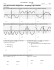### Review Sheet for Second Midterm Mathematics 1300, Calculus 1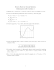### Math 120 Sample questions for Test 2, with answers### Problems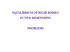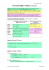### Thursday Mar 5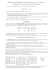### Math 110 Sample Test 4 Answers 1. Do p. 809 #12. Plot the graph of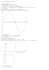### http://iitscholars.com http://iitvidya.com http://iitscholar.com http